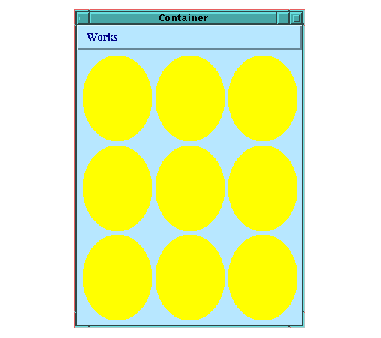### 10.3.2 An example pinboard object

To create your own pinboard objects, the class ``` drawn-pinboard-object``` is provided, which is a ``` pinboard-object``` that accepts a display-callback to display itself. The following example creates a new subclass of ``` drawn-pinboard-object``` that displays an ellipse.

`(defun draw-ellipse-pane (gp pane`
`                             x y`
`                             width height)`
`  (with-geometry pane`
`    (let ((x-radius`
`           (1- (floor %width% 2)))`
`          (y-radius`
`           (1- (floor %height% 2))))`
`      (gp:draw-ellipse `
`       gp`
`       (1+ (+ %x% x-radius))`
`       (1+ (+ %y% y-radius))`
`       x-radius y-radius`
`       :filled t`
`       :foreground `
`       (if (> x-radius y-radius) `
`           :red `
`         :yellow)))))`
` `
`(defclass ellipse-pane`
`     (drawn-pinboard-object)`
`  ()`
`  (:default-initargs`
`   :display-callback 'draw-ellipse-pane`
`   :visible-min-width 50`
`   :visible-min-height 50))`
` `
`(contain`
` (make-instance 'ellipse-pane)`
` :best-width 200`
` :best-height 100)`

Figure 10.7 An ellipse-pane classThe ``` with-geometry``` macro is used to set the size and position, or geometry, of the ellipse drawn by the ``` draw-ellipse-pane``` function. The fill color depends on the radii of the ellipse - try resizing the window to see this. See the LispWorks CAPI Reference Manual for more details of ``` drawn-pinboard-object``` .

Now that you have a new ellipse-pane class, you can create instances of them and place them inside layouts. For instance, the example below creates nine ellipse panes and place them in a three by three grid.

`(contain`
` (make-instance`
`  'grid-layout`
`  :description`
`  (loop for i below 9`
`        collect`
`        (make-instance 'ellipse-pane))`
`  :columns 3)`
` :best-width 300`
` :best-height 400)`

Figure 10.8 Nine ellipse-pane instances in a layoutLispWorks CAPI User Guide (Unix version) - 8 Apr 2005